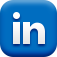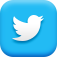# shayla.sawchenko.net## Using Anonymous functions to provide scope

#### 03 December 2013 - Filed under javascript

As you begin to work more with JavaScript, you will inevitably come across 'oddities' when referencing variables; these issues are more than likely caused by scoping and or closure quirks. While scoping and closures are fundamental issues that have been discussed in great detail, there are some subtle nuances that are important to make note of.

I decided to write up this post after I bumped into a StackOverflow question which was asking a fairly common question regarding closures and scopes. While I understood the concept of the problem, I wanted to break it down a little further, and highlight a main conclusion:

You can use anonymous functions to help correctly scope a variable.

## Going through the paces

Let's first describe a series of small examples that may lead you to a case where scoping issues will occur, and then how an anonymous function can save the day.

### Example 1: Building an array of values with a for loop

This is a very common thing to do:

``````var values = [];
for (var i=0;  i < 5; i++) {
values.push(i);
}
console.log(values.toString());
>> 0,1,2,3,4
``````

The array is populated with the values as expected. Since generating things with loops is fun, how about taking it a step further...

### Example 2: Building an array of functions with a for loop

``````var funcs = [];
for (var i=0;  i < 5; i++) {
funcs.push(function() { return i; });
// SPOILER! This does work the way you think it may.
}
``````

Everything looks good right? Let's have a look by calling each of the functions to see their results:

``````var result = [];
for (var j=0;  j < 5; j++) {
result.push(funcs[j]());
}

console.log(result.toString());
>> 5,5,5,5,5
``````

Erm... wat?

## Primitive vs. Object Reference

Given the result in Example 1, you may assume that the result of Example 2 should also be 0,1,2,3,4, however, there is a subtle difference between the two examples causing this discrepancy.

• In Example 1, we are pushing a primitive onto the array (i).

• As it turns out, primitive types are pushed as a copy onto an array - meaning that we have 5 separate allocations in memory, each containing the copied value which is then referenced.
• In Example 2, we are pushing an object onto the array (the function).

• This function references the variable i; as a matter of fact all of the generated functions reference the SAME variable i. Once the for loop is complete, i will have the value 5. Therefore each function is returning the value of 5 when called.

But we want the function to reference the value of i at the time it was created!

## Anonymous functions to the rescue!

If we want to mimic the behaviour of Example 1, then we need to create a copy of i for each function being generated in the for loop. This is where anonymous functions can help!

### What is an anonymous function?

An anonymous function is a function that has no name and is executed immediately:

``````(function() { /* code */ })();
``````

### Anonymous functions have their own scope

Big deal right? At first glance this seems less than useful, however, we can make use of this construct to create a new scope. This gives us the ability to create the variable copy we so desire for Example 2:

### The solution:

``````var funcs = [];
for (var i=0;  i < 5; i++) {
(function(k) {
funcs.push(function() { return k; });
})(i);
}
``````

Here, we create an anonymous function which scopes the variable k and then immediately execute the function, passing in the primative i as the sole parameter. Each function now has access to a locally scoped variable k which stores the value of i at the time the annonomous function was executed. We can verify this with a test:

``````var result = [];
for (var j=0;  j < 5; j++) {
result.push(funcs[j]());
}

console.log(result.toString());
>> 0,1,2,3,4
``````

And there it is - each function returns us the result we desire!

### References:

by Shayla Sawchenko

#### Recent Tweets